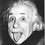# WHY DID BLOCK MOVED ?

TWO EQUALS...YET NOT!! I want to discuss why a tensional force on a block equal to the weight of block makes it move..though UNIFORMLY.

Suppose the block moves height h,by pulling the string in the upward direction, it gains a potential energy,thus we need to increase the force to move it in uniformly, thus, every fraction of second we need to increase the force applied.Now we cannot say that inertia keeps it moving in the required direction.So a force,GREATER THAN the weight force must be applied to keep it moving in upward direction. As my question was, why do we equate the force T to m*g although the block moved uniformly.Note by Aman Kumar
6 years, 9 months ago

This discussion board is a place to discuss our Daily Challenges and the math and science related to those challenges. Explanations are more than just a solution — they should explain the steps and thinking strategies that you used to obtain the solution. Comments should further the discussion of math and science.

When posting on Brilliant:

• Use the emojis to react to an explanation, whether you're congratulating a job well done , or just really confused .
• Ask specific questions about the challenge or the steps in somebody's explanation. Well-posed questions can add a lot to the discussion, but posting "I don't understand!" doesn't help anyone.
• Try to contribute something new to the discussion, whether it is an extension, generalization or other idea related to the challenge.

MarkdownAppears as
*italics* or _italics_ italics
**bold** or __bold__ bold
- bulleted- list
• bulleted
• list
1. numbered2. list
1. numbered
2. list
Note: you must add a full line of space before and after lists for them to show up correctly
paragraph 1paragraph 2

paragraph 1

paragraph 2

[example link](https://brilliant.org)example link
> This is a quote
This is a quote
    # I indented these lines
# 4 spaces, and now they show
# up as a code block.

print "hello world"
# I indented these lines
# 4 spaces, and now they show
# up as a code block.

print "hello world"
MathAppears as
Remember to wrap math in $$ ... $$ or $ ... $ to ensure proper formatting.
2 \times 3 $2 \times 3$
2^{34} $2^{34}$
a_{i-1} $a_{i-1}$
\frac{2}{3} $\frac{2}{3}$
\sqrt{2} $\sqrt{2}$
\sum_{i=1}^3 $\sum_{i=1}^3$
\sin \theta $\sin \theta$
\boxed{123} $\boxed{123}$

Sort by:

We equal the force when there is no acceleration or block moving with constant velocity or at rest.

- 6 years, 9 months ago

when the block starts moving upward,it gains up a velocity from 0 to something, thus, it accelerates.

- 6 years, 9 months ago

Hope you understand.

- 6 years, 8 months ago

Initially it do accelerates

- 6 years, 8 months ago

Equal force on a block when it is in rest is simply its mass into acceleration due to gravity( If it is affected by any gravitation). Equal to the force when it is in motion means different. To move the block the force will not be equal but greater than the force exerted on the string by the block or whatever although it will be equal to the force exerted by the moving block if it moves at a constant speed.

- 6 years, 8 months ago

if tension equals the weight.Then net force on block is zero.If initially it is moving then it remain in motion without change in velocity.

- 6 years, 8 months ago

I AM EDITING THE QUESTION

- 6 years, 8 months ago

the ans is my friend.............. ma=T-mg but as it moves uniformly acceleration is ZERO. thus 0=T-mg T=Mg your concepts r not clear my friend pls read from a better source such as H C Verma

- 6 years, 8 months ago